# Language Models for Text Retrieval Cheng Xiang Zhai

• Slides: 47Language Models for Text Retrieval Cheng. Xiang Zhai Department of Computer Science University of Illinois, Urbana-Champaign 1Outline • • General questions to ask about a language model Probabilistic model for text retrieval Document-generation models Query-generation models 2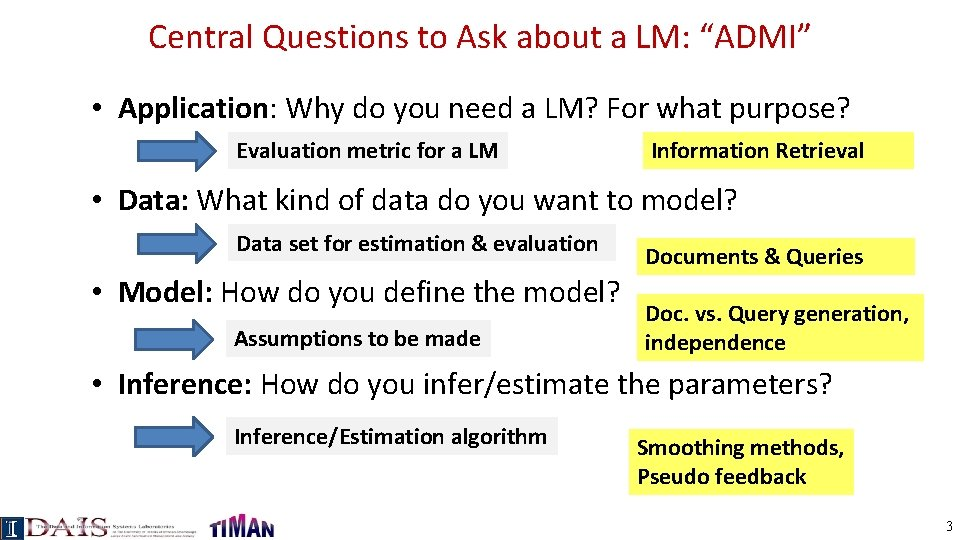Central Questions to Ask about a LM: “ADMI” • Application: Why do you need a LM? For what purpose? Evaluation metric for a LM Information Retrieval • Data: What kind of data do you want to model? Data set for estimation & evaluation • Model: How do you define the model? Assumptions to be made Documents & Queries Doc. vs. Query generation, independence • Inference: How do you infer/estimate the parameters? Inference/Estimation algorithm Smoothing methods, Pseudo feedback 3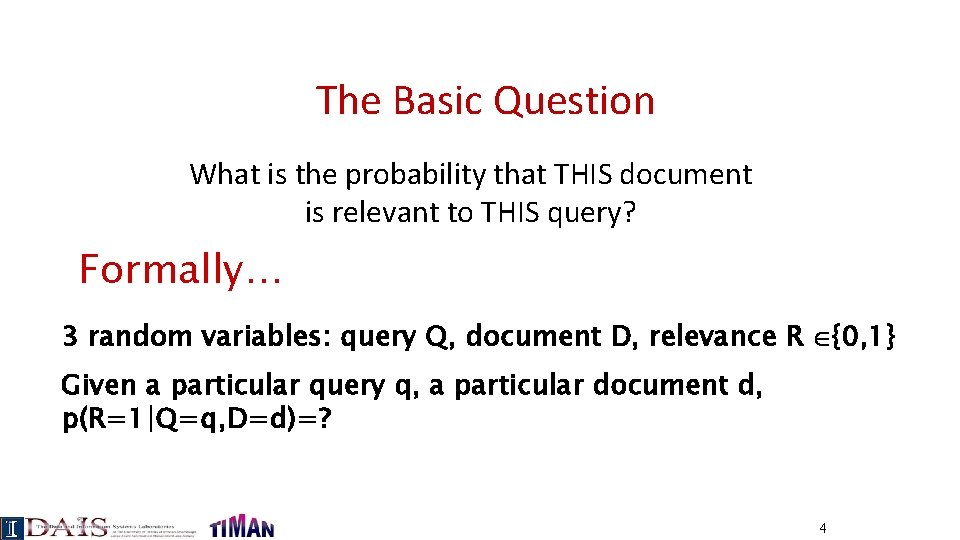The Basic Question What is the probability that THIS document is relevant to THIS query? Formally… 3 random variables: query Q, document D, relevance R {0, 1} Given a particular query q, a particular document d, p(R=1|Q=q, D=d)=? 4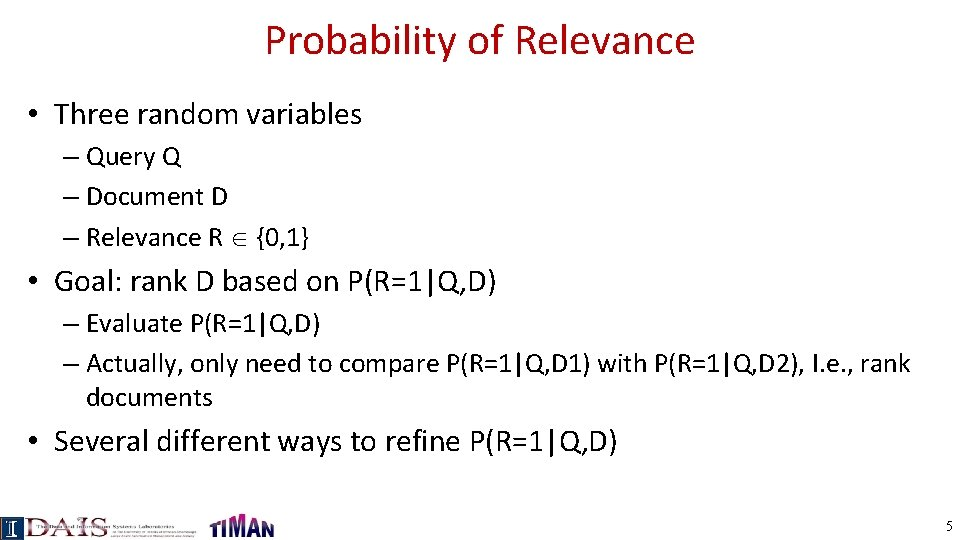Probability of Relevance • Three random variables – Query Q – Document D – Relevance R {0, 1} • Goal: rank D based on P(R=1|Q, D) – Evaluate P(R=1|Q, D) – Actually, only need to compare P(R=1|Q, D 1) with P(R=1|Q, D 2), I. e. , rank documents • Several different ways to refine P(R=1|Q, D) 5Probabilistic Retrieval Models: Intuitions Suppose we have a large number of relevance judgments (e. g. , clickthroughs: “ 1”=clicked; “ 0”= skipped) Query(Q) Doc (D) Q 1 D 1 Q 1 D 2 Q 1 D 3 Q 1 D 4 Q 1 D 5 … Q 1 D 1 Q 1 D 2 Q 1 D 3 Q 2 D 3 Q 3 D 1 Q 4 D 2 Q 4 D 3 … Rel (R) ? 1 1 0 0 1 0 1 1 1 0 We can score documents based on P(R=1|Q 1, D 1)=1/2 P(R=1|Q 1, D 2)=2/2 P(R=1|Q 1, D 3)=0/2 … What if we don’t have (sufficient) search log? We can approximate p(R=1|Q, D)! Different assumptions lead to different models 6Refining P(R=1|Q, D): Generative models • Basic idea – Define P(Q, D|R) – Compute O(R=1|Q, D) using Bayes’ rule Ignored for ranking D • Special cases – Document “generation”: P(Q, D|R)=P(D|Q, R)P(Q|R) – Query “generation”: P(Q, D|R)=P(Q|D, R)P(D|R) 7Document Generation Model of relevant docs for Q Model of non-relevant docs for Q Assume independent attributes A 1…Ak …. (why? ) Let D=d 1…dk, where dk {0, 1} is the value of attribute Ak (Similarly Q=q 1…qk 8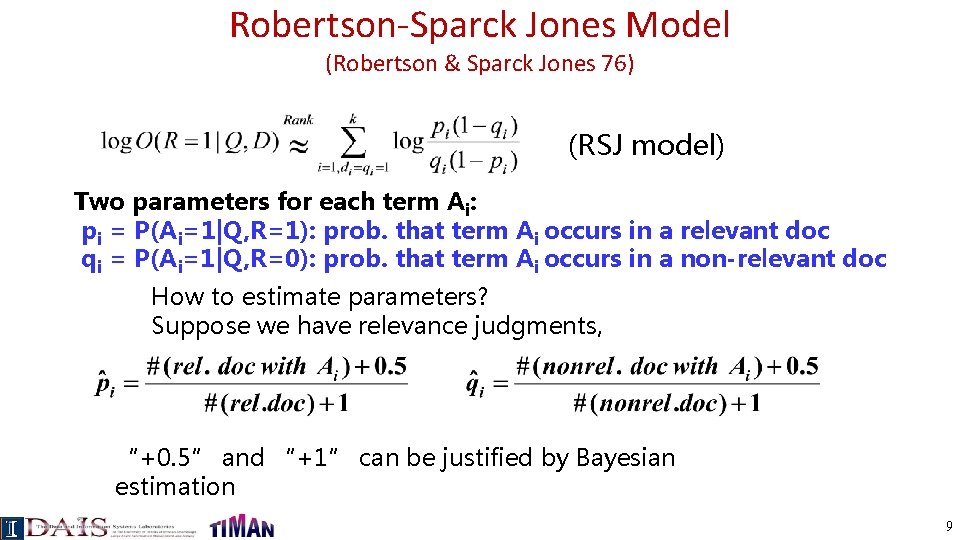Robertson-Sparck Jones Model (Robertson & Sparck Jones 76) (RSJ model) Two parameters for each term Ai: pi = P(Ai=1|Q, R=1): prob. that term Ai occurs in a relevant doc qi = P(Ai=1|Q, R=0): prob. that term Ai occurs in a non-relevant doc How to estimate parameters? Suppose we have relevance judgments, “+0. 5” and “+1” can be justified by Bayesian estimation 9RSJ Model: No Relevance Info (Croft & Harper 79) (RSJ model) How to estimate parameters? Suppose we do not have relevance judgments, - We will assume pi to be a constant - Estimate qi by assuming all documents to be non-relevant N: # documents in collection ni: # documents in which term Ai occurs 10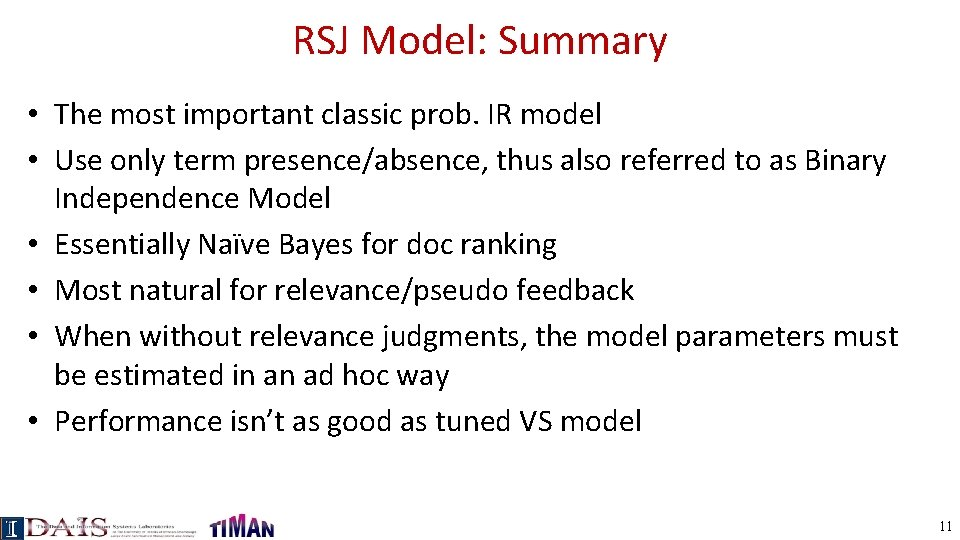RSJ Model: Summary • The most important classic prob. IR model • Use only term presence/absence, thus also referred to as Binary Independence Model • Essentially Naïve Bayes for doc ranking • Most natural for relevance/pseudo feedback • When without relevance judgments, the model parameters must be estimated in an ad hoc way • Performance isn’t as good as tuned VS model 11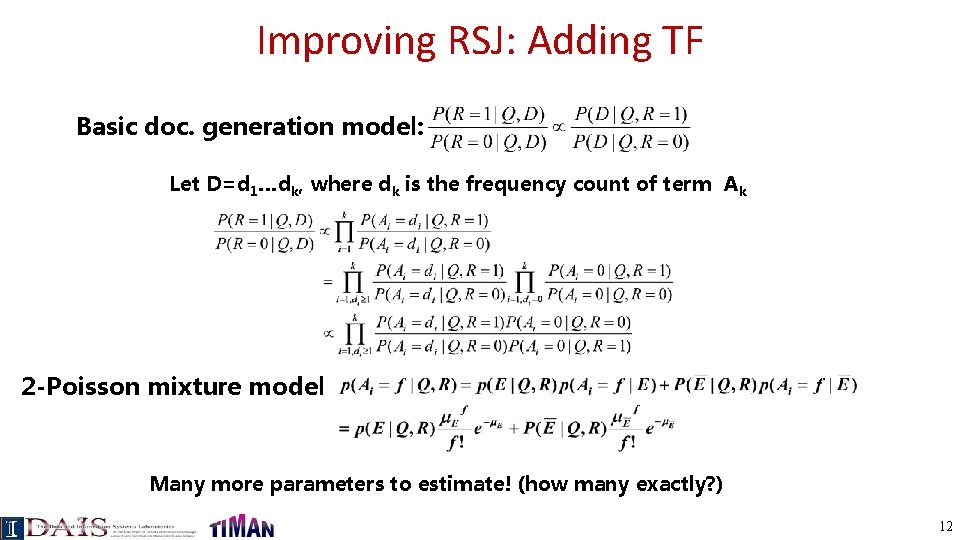Improving RSJ: Adding TF Basic doc. generation model: Let D=d 1…dk, where dk is the frequency count of term Ak 2 -Poisson mixture model Many more parameters to estimate! (how many exactly? ) 12BM 25/Okapi Approximation (Robertson et al. 94) • Idea: Approximate p(R=1|Q, D) with a simpler function that share similar properties • Observations: – log O(R=1|Q, D) is a sum of term weights Wi – Wi= 0, if TFi=0 – Wi increases monotonically with TFi – Wi has an asymptotic limit • The simple function is 13Adding Doc. Length & Query TF • Incorporating doc length – Motivation: The 2 -Poisson model assumes equal document length – Implementation: “Carefully” penalize long doc • Incorporating query TF – Motivation: Appears to be not well-justified – Implementation: A similar TF transformation • The final formula is called BM 25, achieving top TREC performance 14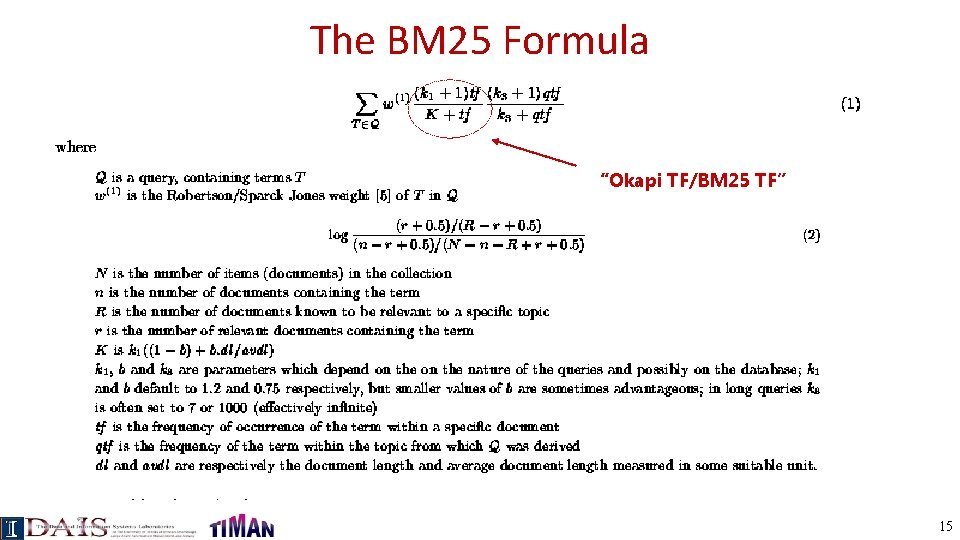The BM 25 Formula “Okapi TF/BM 25 TF” 15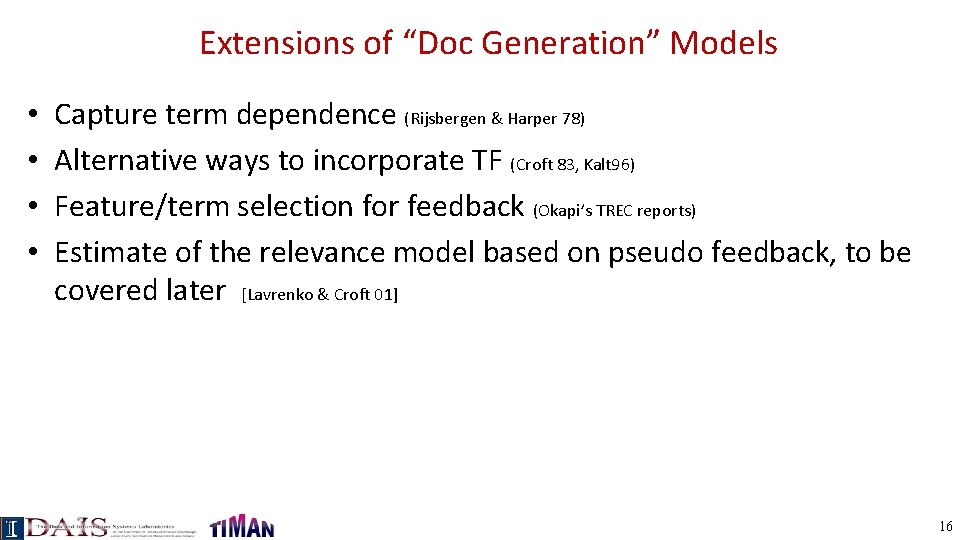Extensions of “Doc Generation” Models • • Capture term dependence (Rijsbergen & Harper 78) Alternative ways to incorporate TF (Croft 83, Kalt 96) Feature/term selection for feedback (Okapi’s TREC reports) Estimate of the relevance model based on pseudo feedback, to be covered later [Lavrenko & Croft 01] 16Query Generation ( Language Models for IR) Query likelihood p(Q| D, R=1) Assuming uniform prior, we have Now, the question is how to compute Document prior ? Generally involves two steps: (1) estimate a language model based on D (2) compute the query likelihood according to the estimated model P(Q|D, R=1) Prob. that a user who likes D would pose query Q. How to estimate it? 17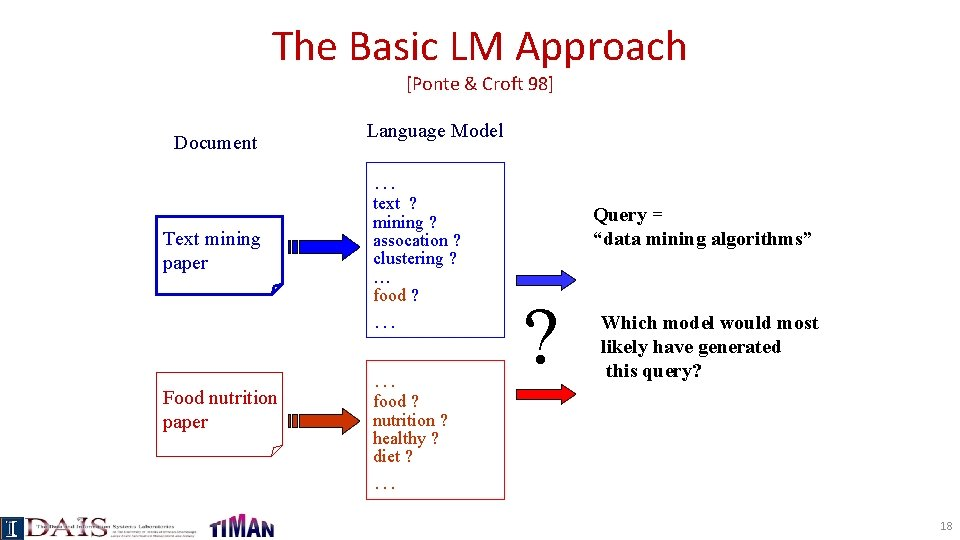The Basic LM Approach [Ponte & Croft 98] Document Language Model … Text mining paper text ? mining ? assocation ? clustering ? … food ? … Food nutrition paper … Query = “data mining algorithms” ? Which model would most likely have generated this query? food ? nutrition ? healthy ? diet ? … 18Ranking Docs by Query Likelihood Doc LM Query likelihood d 1 p(q| d 1) d 2 p(q| d 2) q p(q| d. N) d. N 19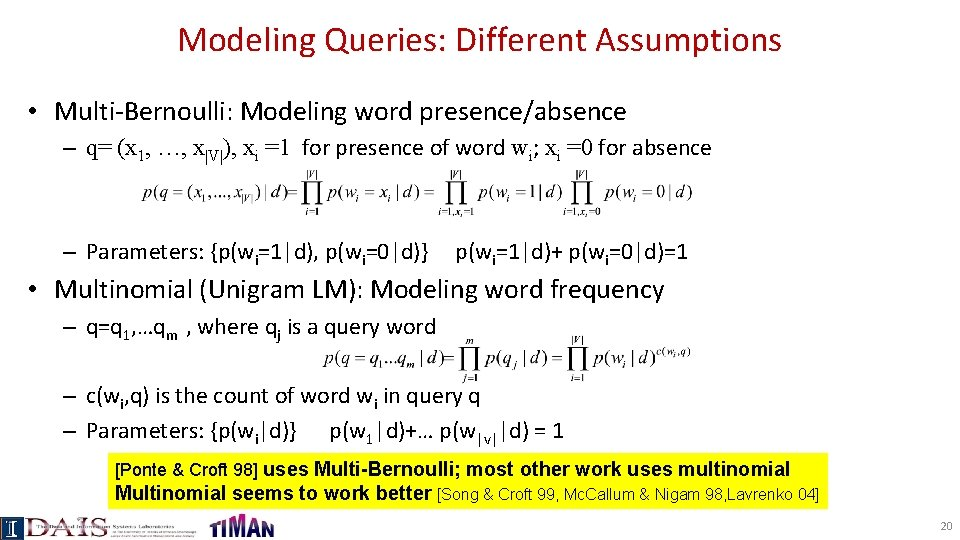Modeling Queries: Different Assumptions • Multi-Bernoulli: Modeling word presence/absence – q= (x 1, …, x|V|), xi =1 for presence of word wi; xi =0 for absence – Parameters: {p(wi=1|d), p(wi=0|d)} p(wi=1|d)+ p(wi=0|d)=1 • Multinomial (Unigram LM): Modeling word frequency – q=q 1, …qm , where qj is a query word – c(wi, q) is the count of word wi in query q – Parameters: {p(wi|d)} p(w 1|d)+… p(w|v||d) = 1 [Ponte & Croft 98] uses Multi-Bernoulli; most other work uses multinomial Multinomial seems to work better [Song & Croft 99, Mc. Callum & Nigam 98, Lavrenko 04] 20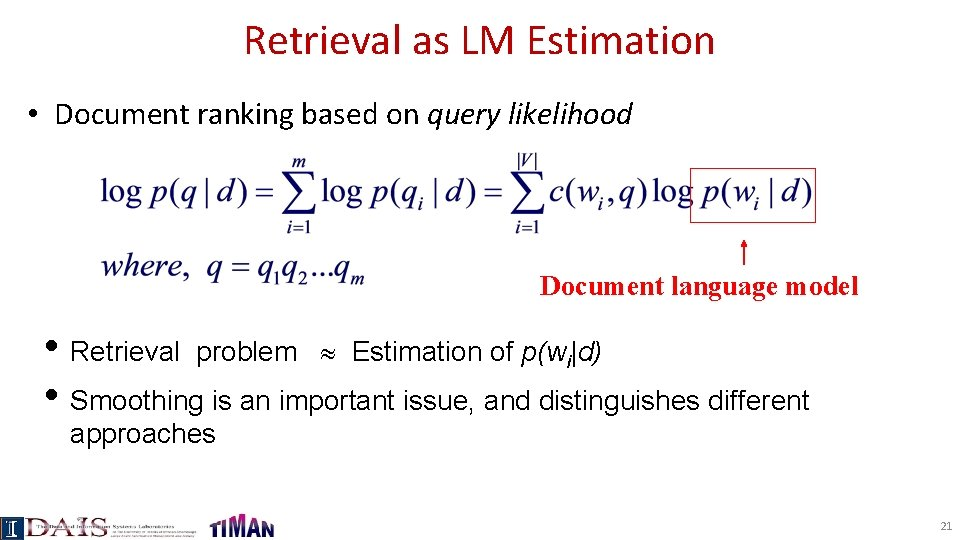Retrieval as LM Estimation • Document ranking based on query likelihood Document language model • Retrieval problem Estimation of p(wi|d) • Smoothing is an important issue, and distinguishes different approaches 21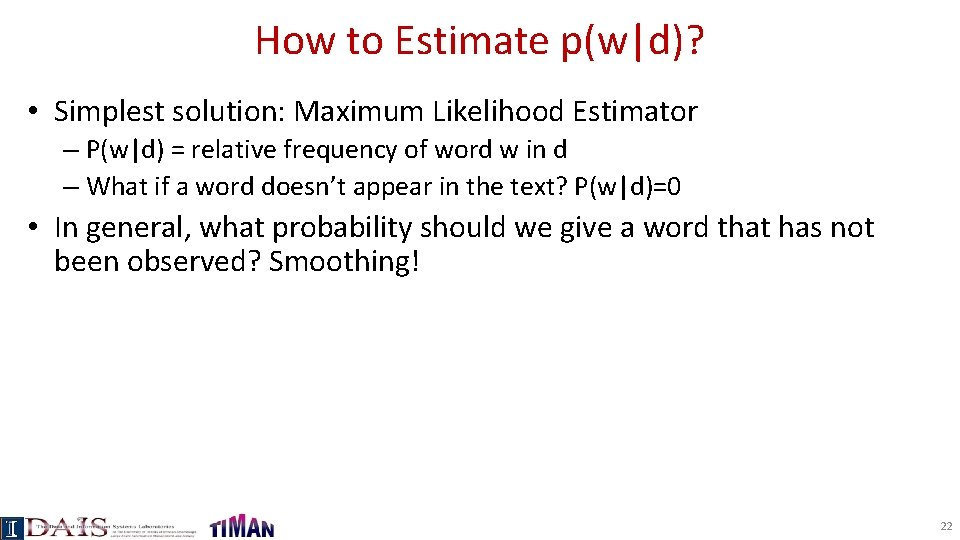How to Estimate p(w|d)? • Simplest solution: Maximum Likelihood Estimator – P(w|d) = relative frequency of word w in d – What if a word doesn’t appear in the text? P(w|d)=0 • In general, what probability should we give a word that has not been observed? Smoothing! 22How to smooth a LM • Key Question: what probability should be assigned to an unseen word? • Let the probability of an unseen word be proportional to its probability given by a reference LM • One possibility: Reference LM = Collection LM Discounted ML estimate Collection language model 23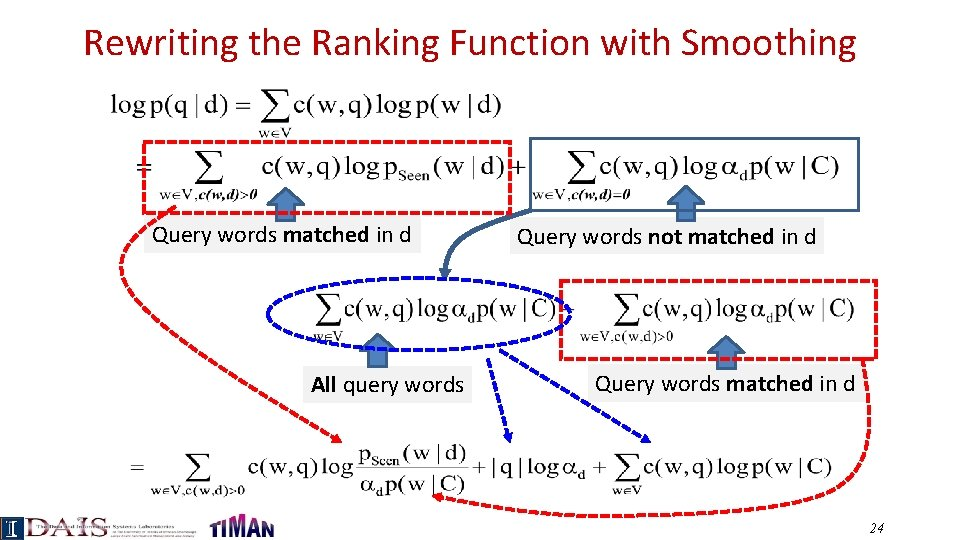Rewriting the Ranking Function with Smoothing Query words matched in d All query words Query words not matched in d Query words matched in d 24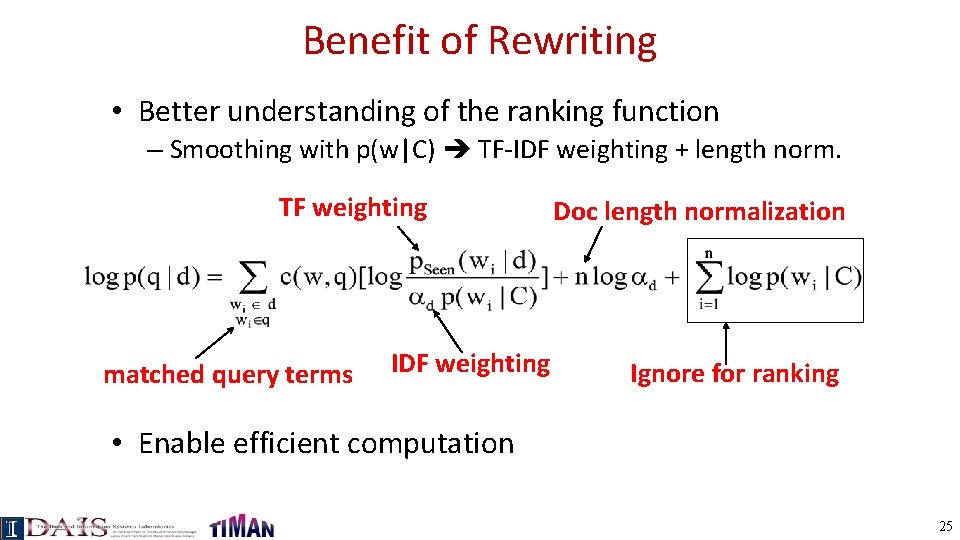Benefit of Rewriting • Better understanding of the ranking function – Smoothing with p(w|C) TF-IDF weighting + length norm. TF weighting matched query terms IDF weighting Doc length normalization Ignore for ranking • Enable efficient computation 25Query Likelihood Retrieval Functions With Jelinek-Mercer (JM): With Dirichlet Prior (DIR): What assumptions have we made in order to derive these functions? Do they capture the same retrieval heuristics (TF-IDF, Length Norm) as a vector space retrieval function? 26So, which method is the best? It depends on the data and the task! Cross validation is generally used to choose the best method and/or set the smoothing parameters… For retrieval, Dirichlet prior performs well… Backoff smoothing [Katz 87] doesn’t work well due to a lack of 2 ndstage smoothing… 27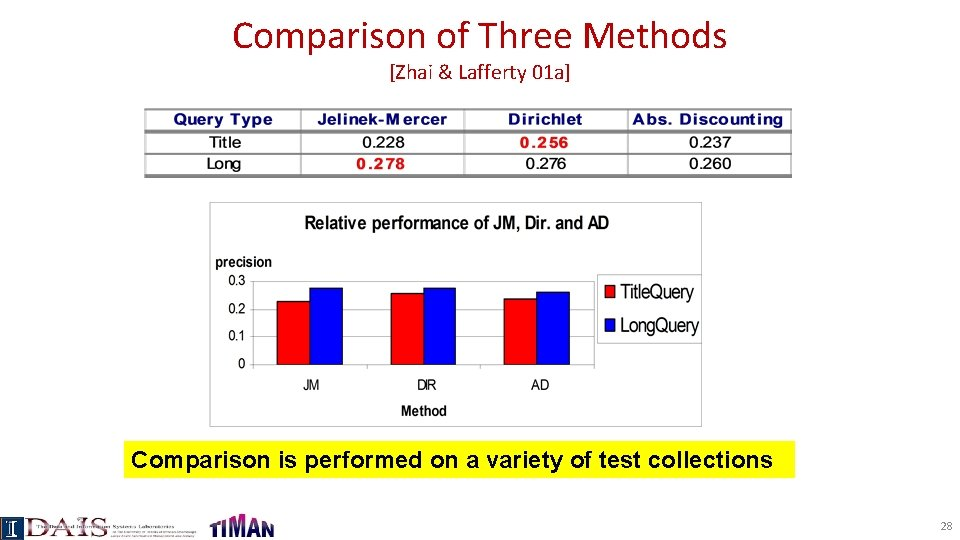Comparison of Three Methods [Zhai & Lafferty 01 a] Comparison is performed on a variety of test collections 28The Dual-Role of Smoothing [Zhai & Lafferty 02] long Verbose queries Keyword queries long short Why does query type affect smoothing sensitivity? 29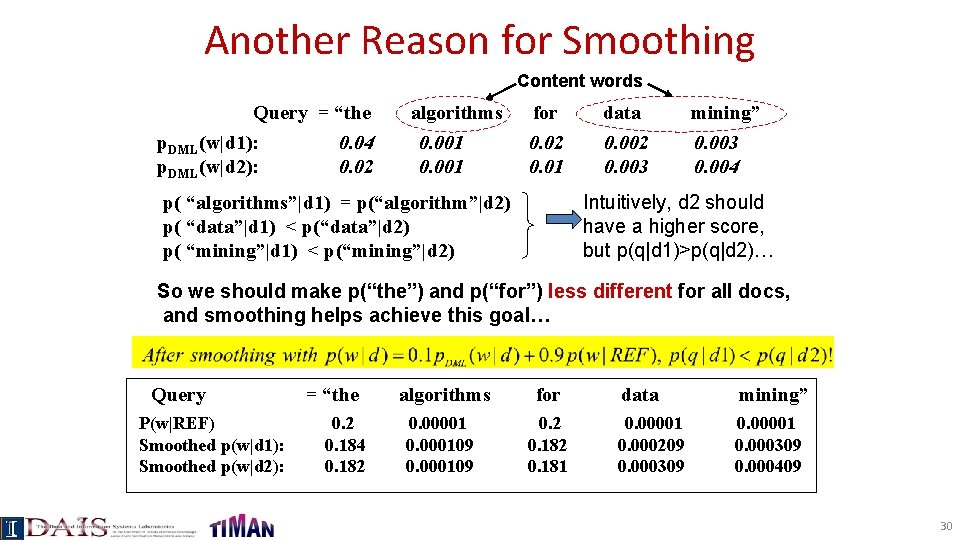Another Reason for Smoothing Content words Query = “the p. DML(w|d 1): 0. 04 p. DML(w|d 2): 0. 02 algorithms 0. 001 for 0. 02 0. 01 data 0. 002 0. 003 mining” 0. 003 0. 004 Intuitively, d 2 should have a higher score, but p(q|d 1)>p(q|d 2)… p( “algorithms”|d 1) = p(“algorithm”|d 2) p( “data”|d 1) < p(“data”|d 2) p( “mining”|d 1) < p(“mining”|d 2) So we should make p(“the”) and p(“for”) less different for all docs, and smoothing helps achieve this goal… Query P(w|REF) Smoothed p(w|d 1): Smoothed p(w|d 2): = “the 0. 2 0. 184 0. 182 algorithms for 0. 00001 0. 000109 0. 2 0. 181 data mining” 0. 00001 0. 000209 0. 000309 0. 00001 0. 000309 0. 000409 30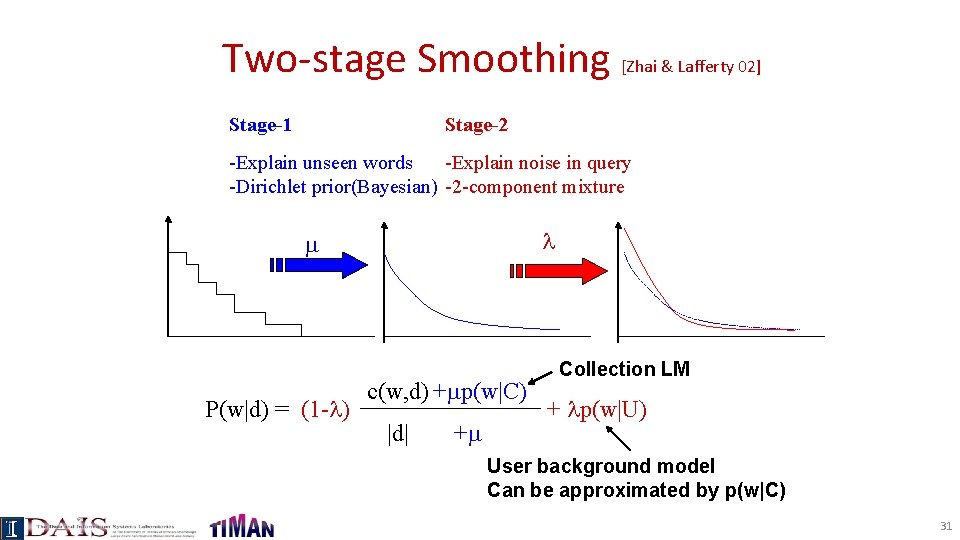Two-stage Smoothing [Zhai & Lafferty 02] Stage-1 Stage-2 -Explain unseen words -Explain noise in query -Dirichlet prior(Bayesian) -2 -component mixture P(w|d) = (1 - ) c(w, d) + p(w|C) |d| + Collection LM + p(w|U) User background model Can be approximated by p(w|C) 31Estimating using leave-one-out [Zhai & Lafferty 02] w 1 P(w 1|d- w 1) log-likelihood Leave-one-out w 2 P(w 2|d- w 2) Maximum Likelihood Estimator . . . wn P(wn|d- wn) Newton’s Method 32Why would “leave-one-out” work? 20 word by author 1 abc ab c d d abc cd d d ab ab cd d e cd e Suppose we keep sampling and get 10 more words. Which author is likely to “write” more new words? Now, suppose we leave “e” out… doesn’t have to be big 20 word by author 2 abc ab c d d abe cb e f acf fb ef aff abef cdc db ge f s must be big! more smoothing The amount of smoothing is closely related to the underlying vocabulary size 33Estimating using Mixture Model [Zhai & Lafferty 02] Stage-2 Stage-1 d 1 P(w|d 1) (1 - )p(w|d 1)+ p(w|U) . . . …. . . d. N P(w|d. N) 1 N Query Q=q 1…qm (1 - )p(w|d. N)+ p(w|U) Estimated in stage-1 Maximum Likelihood Estimator Expectation-Maximization (EM) algorithm 34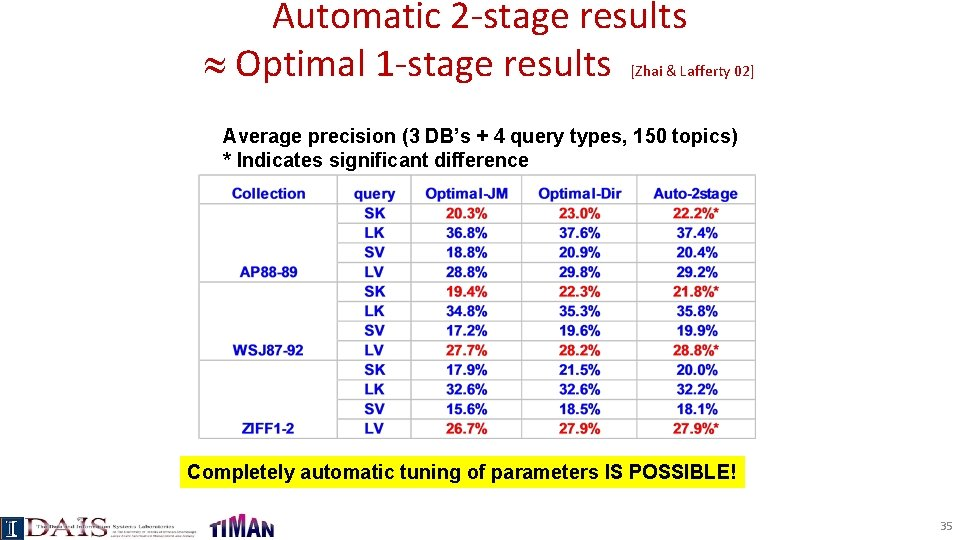Automatic 2 -stage results Optimal 1 -stage results [Zhai & Lafferty 02] Average precision (3 DB’s + 4 query types, 150 topics) * Indicates significant difference Completely automatic tuning of parameters IS POSSIBLE! 35Feedback and Doc/Query Generation Rel. doc model Classic Prob. Model Non. Rel. doc model Query likelihood (“Language Model”) Parameter Estimation (q 1, d 1, 1) (q 1, d 2, 1) (q 1, d 3, 1) (q 1, d 4, 0) (q 1, d 5, 0) (q 3, d 1, 1) (q 4, d 1, 1) (q 5, d 1, 1) (q 6, d 2, 1) (q 6, d 3, 0) “Rel. query” model P(D|Q, R=1) P(D|Q, R=0) P(Q|D, R=1) Initial retrieval: - query as rel doc vs. doc as rel query - P(Q|D, R=1) is more accurate Feedback: - P(D|Q, R=1) can be improved for the current query and future doc - P(Q|D, R=1) can also be improved, but for current doc and future query Query-based feedback Doc-based feedback 36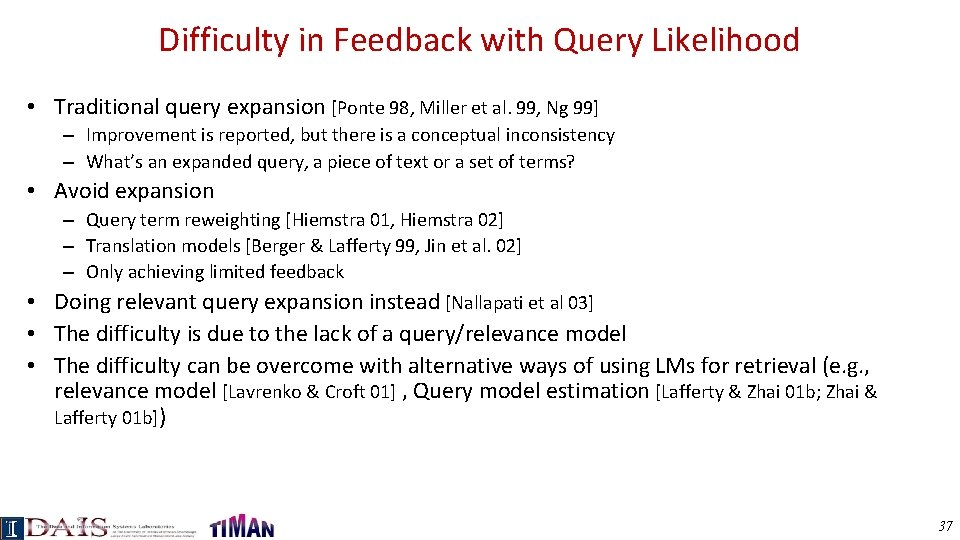Difficulty in Feedback with Query Likelihood • Traditional query expansion [Ponte 98, Miller et al. 99, Ng 99] – Improvement is reported, but there is a conceptual inconsistency – What’s an expanded query, a piece of text or a set of terms? • Avoid expansion – Query term reweighting [Hiemstra 01, Hiemstra 02] – Translation models [Berger & Lafferty 99, Jin et al. 02] – Only achieving limited feedback • Doing relevant query expansion instead [Nallapati et al 03] • The difficulty is due to the lack of a query/relevance model • The difficulty can be overcome with alternative ways of using LMs for retrieval (e. g. , relevance model [Lavrenko & Croft 01] , Query model estimation [Lafferty & Zhai 01 b; Zhai & Lafferty 01 b]) 37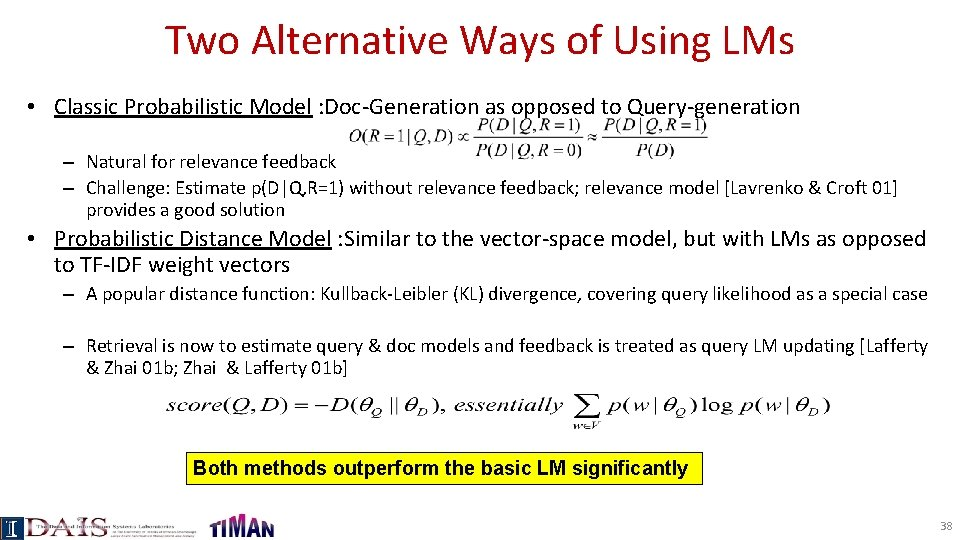Two Alternative Ways of Using LMs • Classic Probabilistic Model : Doc-Generation as opposed to Query-generation – Natural for relevance feedback – Challenge: Estimate p(D|Q, R=1) without relevance feedback; relevance model [Lavrenko & Croft 01] provides a good solution • Probabilistic Distance Model : Similar to the vector-space model, but with LMs as opposed to TF-IDF weight vectors – A popular distance function: Kullback-Leibler (KL) divergence, covering query likelihood as a special case – Retrieval is now to estimate query & doc models and feedback is treated as query LM updating [Lafferty & Zhai 01 b; Zhai & Lafferty 01 b] Both methods outperform the basic LM significantly 38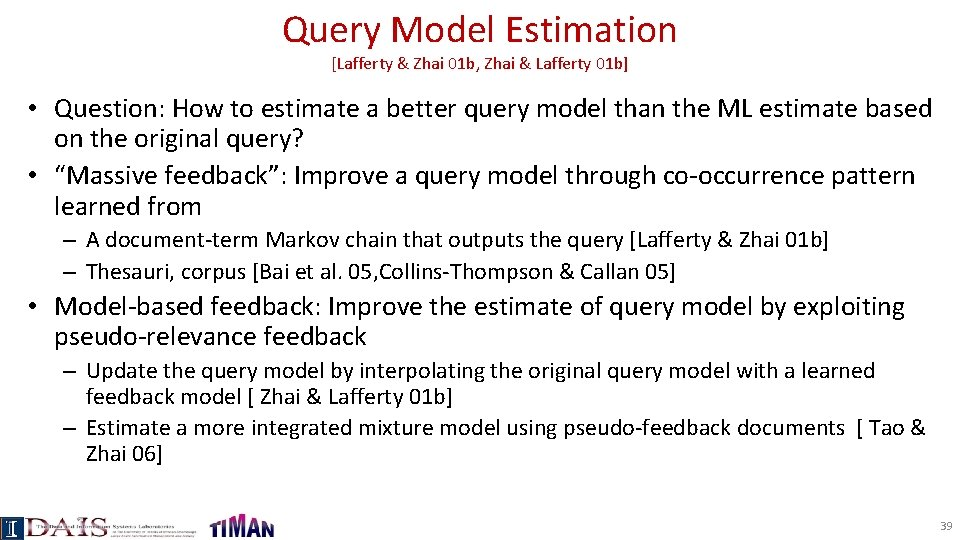Query Model Estimation [Lafferty & Zhai 01 b, Zhai & Lafferty 01 b] • Question: How to estimate a better query model than the ML estimate based on the original query? • “Massive feedback”: Improve a query model through co-occurrence pattern learned from – A document-term Markov chain that outputs the query [Lafferty & Zhai 01 b] – Thesauri, corpus [Bai et al. 05, Collins-Thompson & Callan 05] • Model-based feedback: Improve the estimate of query model by exploiting pseudo-relevance feedback – Update the query model by interpolating the original query model with a learned feedback model [ Zhai & Lafferty 01 b] – Estimate a more integrated mixture model using pseudo-feedback documents [ Tao & Zhai 06] 39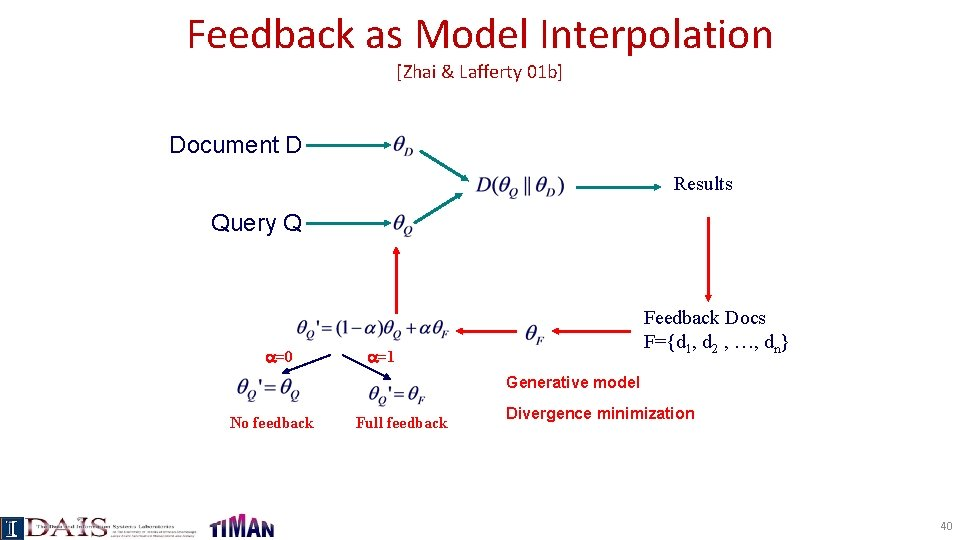Feedback as Model Interpolation [Zhai & Lafferty 01 b] Document D Results Query Q =0 Feedback Docs F={d 1, d 2 , …, dn} =1 Generative model No feedback Full feedback Divergence minimization 40F Estimation Method I: Generative Mixture Model Background words P(w| C) w P(source) 1 - F={D 1, …, Dn} Topic words P(w| ) w Maximum Likelihood The learned topic model is called a “parsimonious language model” in [Hiemstra et al. 04] 41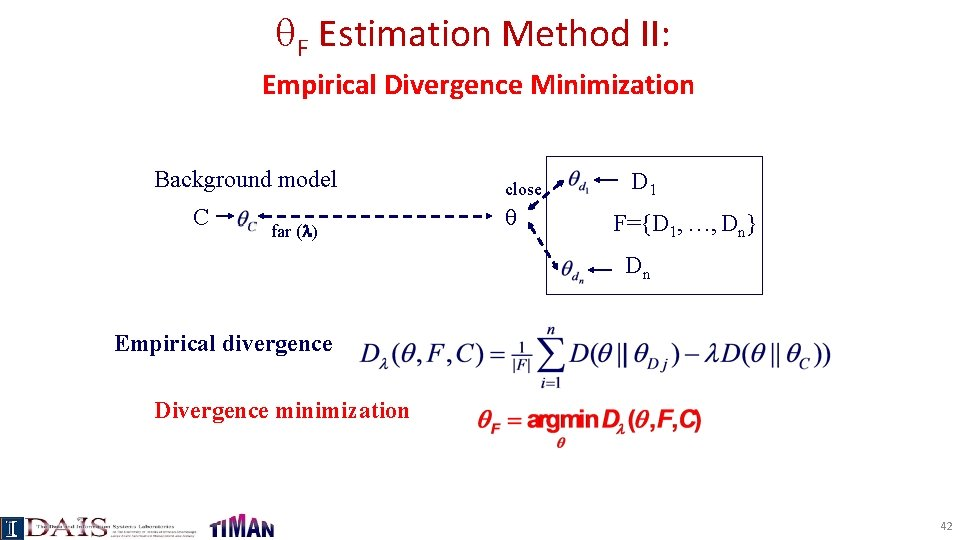F Estimation Method II: Empirical Divergence Minimization Background model C far ( ) close D 1 F={D 1, …, Dn} Dn Empirical divergence Divergence minimization 42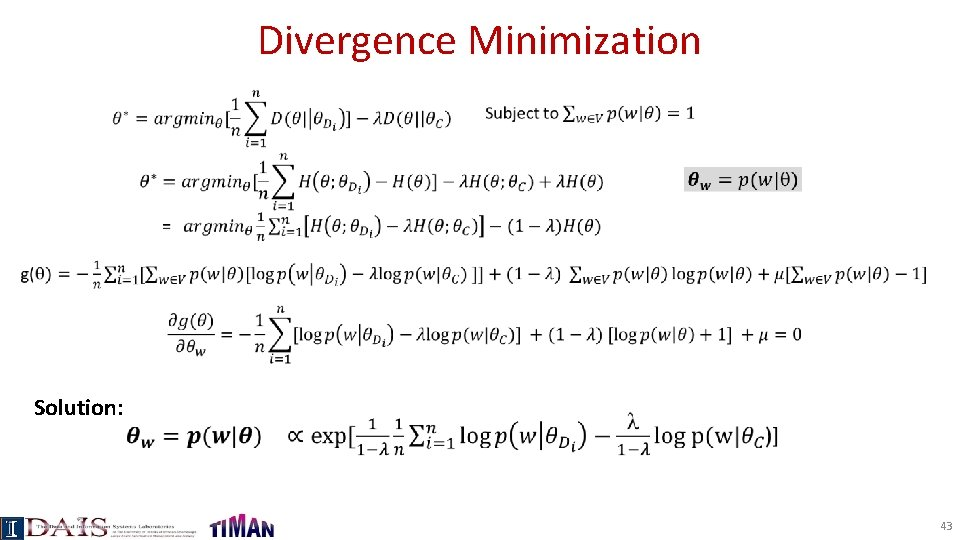Divergence Minimization Solution: 43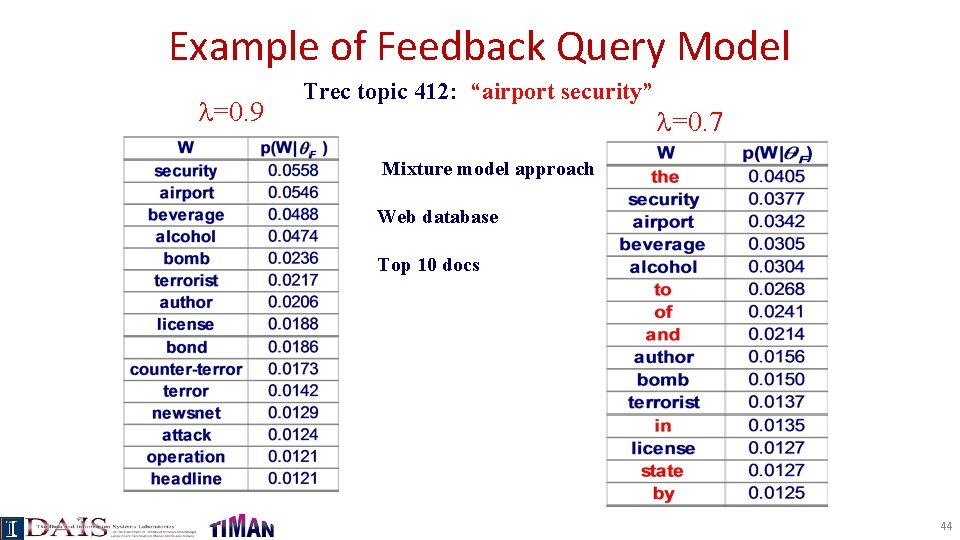Example of Feedback Query Model =0. 9 Trec topic 412: “airport security” =0. 7 Mixture model approach Web database Top 10 docs 44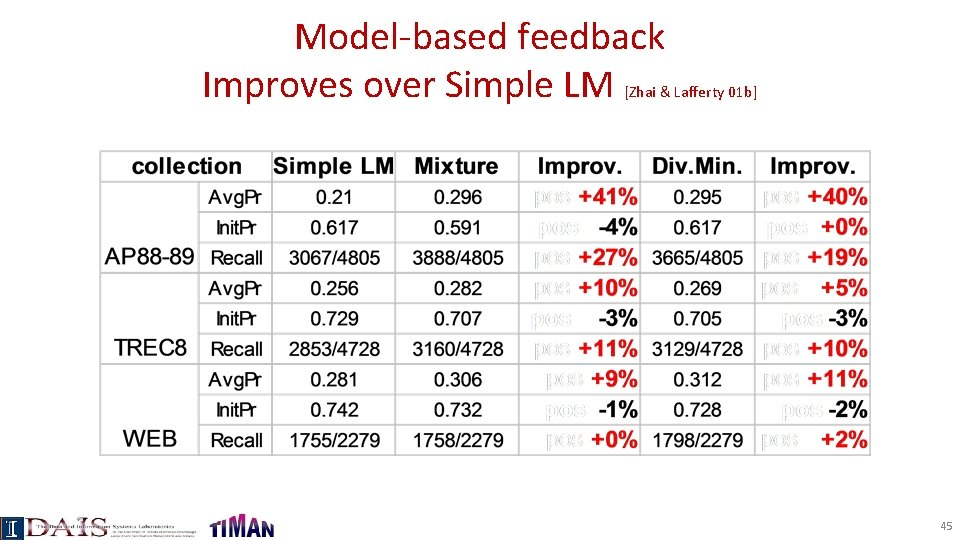Model-based feedback Improves over Simple LM [Zhai & Lafferty 01 b] 45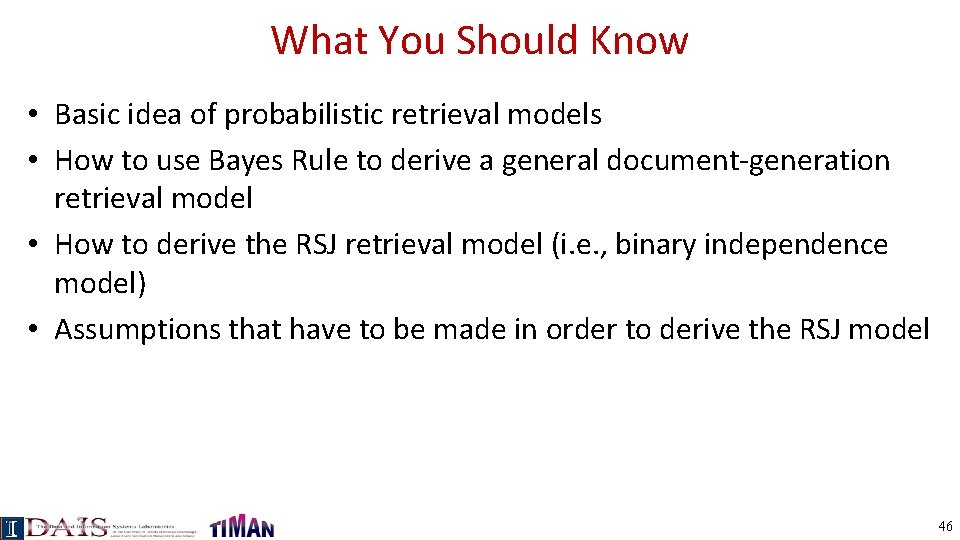What You Should Know • Basic idea of probabilistic retrieval models • How to use Bayes Rule to derive a general document-generation retrieval model • How to derive the RSJ retrieval model (i. e. , binary independence model) • Assumptions that have to be made in order to derive the RSJ model 46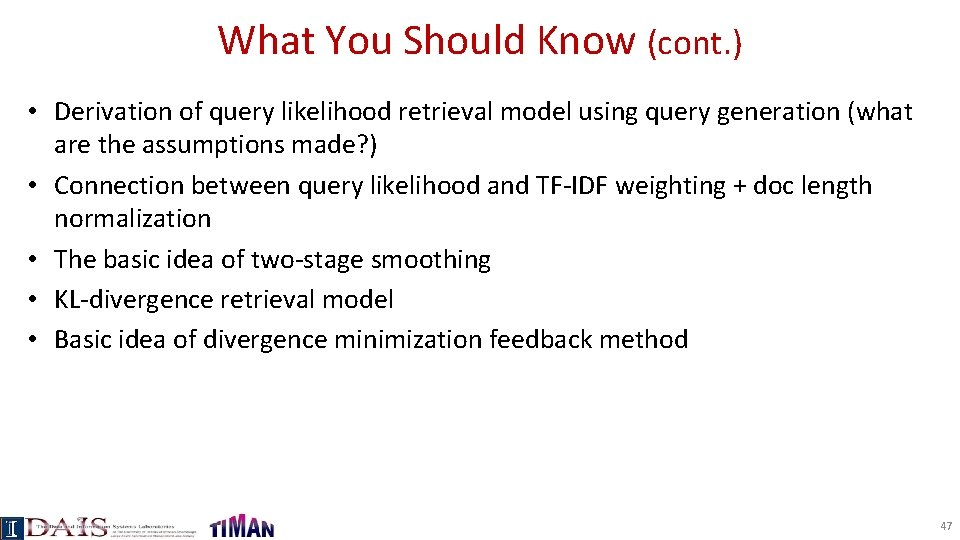What You Should Know (cont. ) • Derivation of query likelihood retrieval model using query generation (what are the assumptions made? ) • Connection between query likelihood and TF-IDF weighting + doc length normalization • The basic idea of two-stage smoothing • KL-divergence retrieval model • Basic idea of divergence minimization feedback method 47